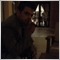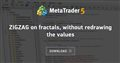# help with call 2 indicator in order make my ea11

Hi!

I saw this indicator(ZIGZAG on fractals, without redrawing the values - https://www.mql5.com/en/code/10022) and I would like to get the last 2 values for an EA.

The solution that I found only return one value for both cases (2147483647) using iCustom.

this number means no fractal if we got price how we identify if we got upper fractal or down fractal

and same here

how i call it on ea and indentify that price represent red or blue line

thanksZIGZAG on fractals, without redrawing the values
• www.mql5.com
Zigzag lines are built on fractals26060

1. theodosis kindinis:

The solution that I found only return one value for both cases (2147483647) using iCustom.

this number means no fractal if we got price how we identify if we got upper fractal or down fractal

Yes, EMPTY_VALUE does mean no fractal at that bar. There is never a fractal at bars zero through two. Bar three maybe. Find the last two bars with a non-EMPTY_VALUE.

2. theodosis kindinis: how we identify if we got upper fractal or down fractal

Makes no sense. You specified which buffer to look at with the iCustom call.